Seaborn distplot lets you show a histogram with a line on it. This can be shown in all kinds of variations. We use seaborn in combination with matplotlib, the Python plotting module.

A distplot plots a univariate distribution of observations. The distplot() function combines the matplotlib hist function with the seaborn kdeplot() and rugplot() functions.

Related course: Matplotlib Examples and Video Course

## Example

### Distplot example

The plot below shows a simple distribution. It creats random values with random.randn().
This will work if you manually define values too.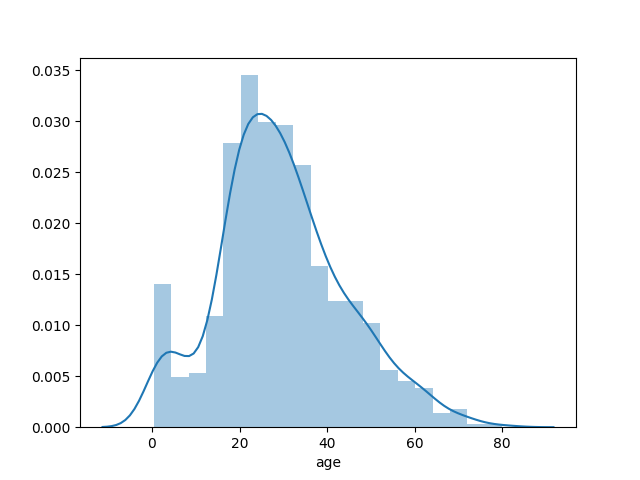### Distplot examples

You can show all kinds of variations of the distplot. We use the subplot() method from the pylab module to show 4 variations at once.

By changing the parameters in the distplot() method you can create totally different views. You can play around with these parameters to change color, orientation and more.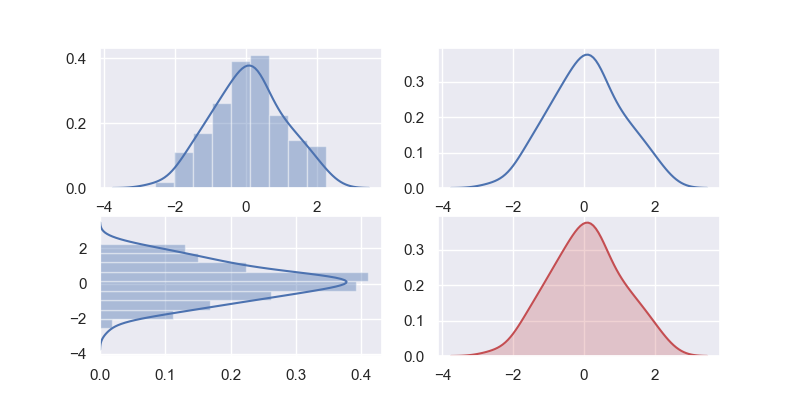### Seaborn dist

You an show a standard dataset from seaborn in histogram too.
This is qutie a large dataset so only take one column.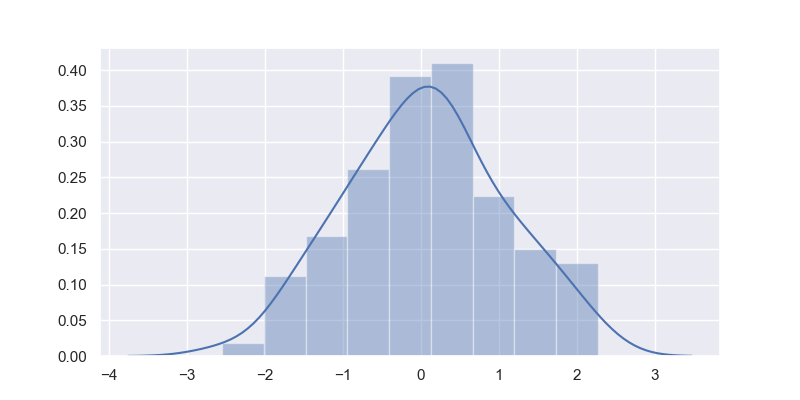### Distplot bins

If you want to change the number of bins or hide the line, that’s possble too.
When calling the method distplot9) you can pass the number of bins and tell the line (kde) to be invisible.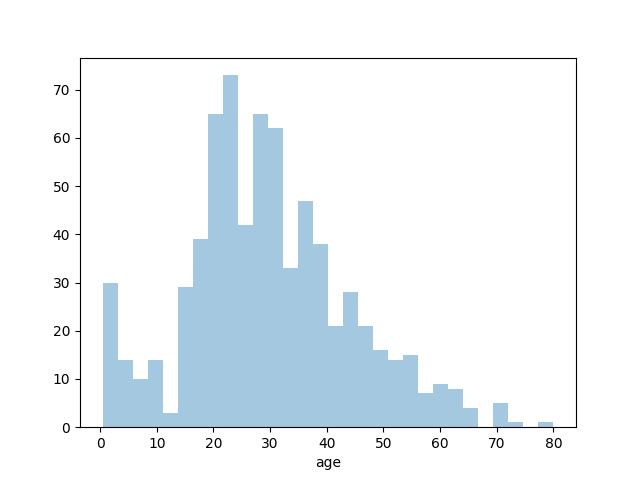### Seaborn different plots

The example below shows some other distribution plots examples. You an activate a grid with the grid(True) method call.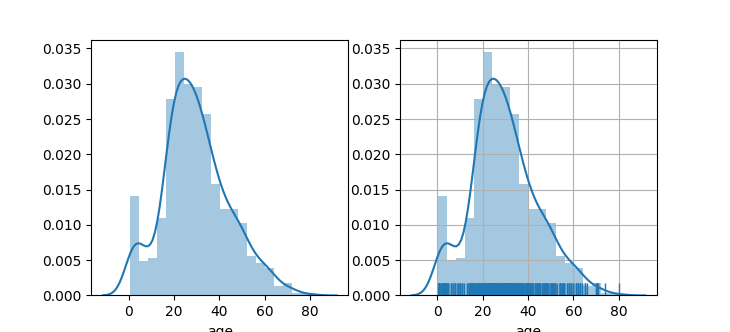If you are new to matplotlib, then I highly recommend this course.